Courses

# Twisting of Solid Open Section Civil Engineering (CE) Notes | EduRev

## Civil Engineering (CE) : Twisting of Solid Open Section Civil Engineering (CE) Notes | EduRev

The document Twisting of Solid Open Section Civil Engineering (CE) Notes | EduRev is a part of the Civil Engineering (CE) Course Advanced Solid Mechanics - Notes, Videos, MCQs & PPTs.
All you need of Civil Engineering (CE) at this link: Civil Engineering (CE)

Twisting of solid open section

In this section, as in the previous, we consider a bar subjected to twisting moments at its ends. The bar axis is straight and the shape of the cross section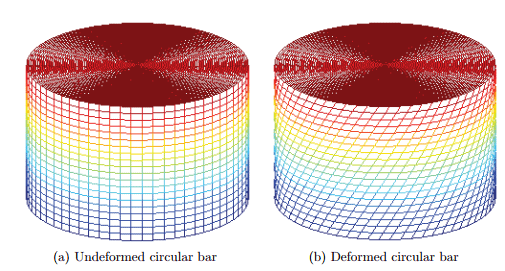Figure 9.8: Deformation of a bar with circular cross section subjected to end torsion

is constant along the axis. The bar is assumed to have a solid cross section, i.e, a simply connected domain. A domain is said to be simply connected if any closed curve in the domain can be shrunk to a point in the domain itself, without leaving the domain. A domain which is not simply connected is said to be multiply connected. We shall analyze multiply connected domains in the next section.

Though the problem formulation does not assume any specific shape for the cross section, the displacement and stress field obtained are cross section specific. Let us assume that the boundary of the cross section is defined by a function, f(x, y) = 0. This function for a ellipse centered about the origin and major and minor axis oriented about the ex and ey directions would be, f(x, y) = x 2/a2+y 2/b2−1. Consequently, for the body is assumed to occupy a region in Euclidean point space, defined by B = {(x, y, z)|f(x, y) ≤ 0, 0 ≤ Z ≤ L}, where L is a constant. Here we are assuming that the bar is a solid cross section with the cross section having only one surface, f(x, y) = 0 denoted by ∂A. To be more precise, the cross section is simply connected. Since, we are interested in the case where in the bar is subjected to pure end torsional moment, the traction boundary conditions are: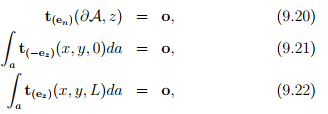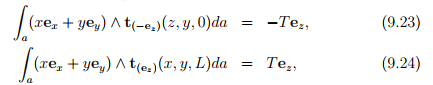where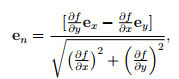(9.25)

and T is a constant. Here en is obtained such that it is in the xy plane, perpendicular to grad(f) and is a unit vector. Recognize that grad(f) gives the tangent vector to the cross section for any point in ∂A. The orientation of eand grad(f) is as shown in figure 9.9. Further, since one end of this body is assumed to be fixed against twisting but free to displace axially and the other end free to displace in all directions, the displacement boundary conditions for this problem is,

u(x, y, 0) = uz(x, y)ez,                     (9.26)

where uz is any function of x and y. Here we have assumed that the surface of the body defined by z = 0 is fixed against twisting but free to displace axially and the other surfaces are free to displace in all directions.

In a solid cross section, as a result of a twist, each cross section undergoes a rotational displacement about the z axis. For any two cross sections the relative angle of rotation is called the angle of twist between the two sections. Let ∆β be the angle of twist for two cross sections at distance ∆z and Ω be the angle of twist per unit length of the bar, then

∆β = Ω(∆z).                         (9.27)

Since the conditions are same for all cross sections, the above equation applies to any two cross sections at a distance ∆z along the bar length. Thus, Ω is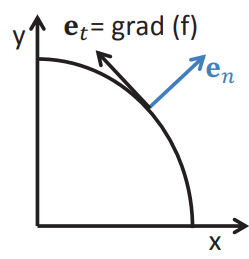Figure 9.9: Boundary conditions at the surface of a bar subjected to end torsion

a constant along the bar length. Also, since at z = 0, β = 0, by virtue of the surface defined by z = 0 being fixed against rotation, the angle of twist, β for a cross sections at distance z from the surface defined by z = 0 is

β = Ωz.                          9.28)

Consider a cross section at a distance z from the fixed end. In that section consider a point A with coordinates (x, y, z). Assuming the origin of the coordinate system to be located at the axis of twisting, let r denote the radial distance of the point A from the origin and let θ denote the angle that this radial line makes with the ex, as shown in the figure 9.10. Let A move to A' due to twisting of the bar, as shown in the figure 9.10. The arc length AA' would be equal to rβ, where β is the angle of twist at the cross section and is related to the angle of twist per unit length through (9.28). Hence, the arc length AA' would be,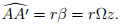(9.29)

Since, the angle of twist β is small, we approximate the secant length, i.e., the length of the straight line between AA' with its arc length. Consequently, the x component of the displacement of A is given by,(9.30)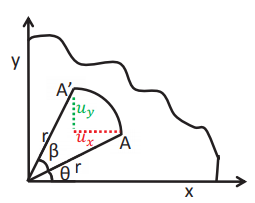Figure 9.10: Displacements in the cross section of a bar subjected to end twisting moment

where we have used (9.29) and the relation that y = r sin(θ). Similarly, the y component of the displacement of A is,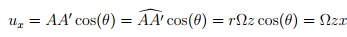(9.31)

where as before we used (9.29) and the relation x = r cos(θ). Since, there is no restraint against displacement along ez direction at any section, all cross sections is assumed to undergo the same displacement along the z direction and hence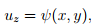(9.32)

where ψ is a function of x and y only. That is, we have assumed that the z component of the displacement is independent of the axial location of the section. Presence of this z component of the displacement is the characteristic of open sections. It is due to this z component of the displacement the plane section distorts and is said to warp. Consequently, ψ is called Saint-Venant’s warping function. Thus, the displacement field for a bar subjected to twisting moments at the end and is free to warp is given by,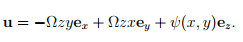(9.33)

It can be easily verified that the above displacement field (9.33) satisfies the displacement boundary condition (9.26).

Substituting equation (9.33) in the strain-displacement equation (7.1), the Cartesian components of the strain are computed to be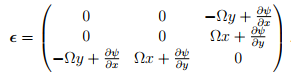(9.34)

For this state of strain, the corresponding Cartesian components of the stress are obtained from Hooke’s law (7.2) as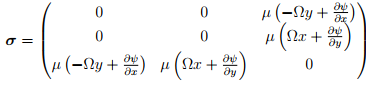(9.35)

For this stress state, (9.35) to be possible in a body in static equilibrium, without any body force acting on it, it should satisfy the balance of linear momentum equations (7.6) which requires that,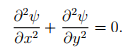(9.36)

Now, we have to find ψ such that equation (9.36) holds along with the prescribed traction boundary conditions (9.20) through (9.24). Boundary condition (9.20) requires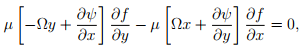(9.37)

for (x, y) ∈ ∂A. Both the boundary conditions (9.21) and (9.22) yield the following restriction on ψ,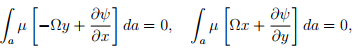(9.38)

On assuming that the bar is homogeneous and appealing to Green’s theorem (section 2.9.3), equation (9.38) reduces to,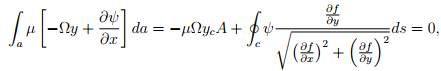(9.39)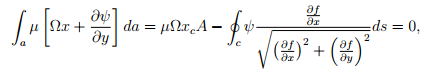(9.40)

where (xc, yc) is the coordinates of the centroid of the cross section. On assuming that the axis of twisting coincides with the centroid and further requiring that the origin of the coordinate system coincide with the centroid of the cross section, equations (9.39) and (9.40) requires,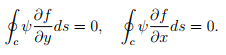(9.42)

Finally, the boundary conditions (9.23) and (9.24) require that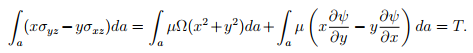(9.42)

Recognizing that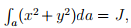, polar moment of inertia equation (9.42) can be simplified as,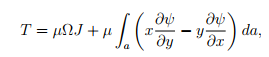(9.43)

where we have used the assumption already made that the bar is homogeneous. For many cross sections,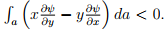Thus, the torsional stiffness1 which would be µJ, in the absence of warping (i.e., ψ = 0), decreases due to warping (i.e., when ψ 6= 0). Hence, warping is said to decrease the torsional stiffness of the cross section. Equation (9.43) is used find Ω. Experience has shown that it is difficult to find ψ that satisfies the governing equation (9.36) along with the boundary conditions (9.37) and (9.41). Hence, we recast the problem using Prandtl stress function.

Stress function formulation Defining a differentiable function, φ = φˆ(x, y) called the Prandtl stress function, we relate the components of the stress to this stress function as,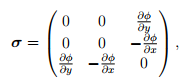(9.44)

 1 Torsional stiffness is defined as the torque required to cause a unit angle of twist per unit length.

so that the equilibrium equations (7.6) are satisfied for any choice of φ. Now, one can proceed in one of two ways. Follow the standard approach and find the strain corresponding to the stress state (9.44), substitute the same in the compatibility conditions and find the governing equation that φ should satisfy. Here we obtain the same governing equation through an alternate approach. Equating the stress states (9.35) and (9.44) as it represents for the same boundary value problem, we obtain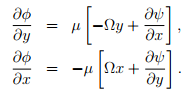(9.45 & 9.46)

Differentiating equation (9.45) with respect to y and equation (9.46) with respect to x and adding the resulting equations, we obtain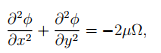(9.47)

where we have made use of the requirement that ∂ 2ψ ∂x∂y = ∂ 2ψ ∂y∂x . By virtue of the stress function being smooth enough so that ∂ 2φ ∂x∂y = ∂ 2φ ∂y∂x , the governing equation for ψ (9.36) is trivially satisfied. The boundary condition given in equation (9.37) in terms of the warping function, ψ is rewritten in terms of the Prandtl stress function, φ using equations (9.45) and (9.46) as,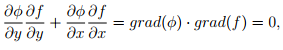(9.48)

for (x, y) ∈ ∂A. Since, grad(f) ≠ o and its direction changes with the location on the boundary of the cross section, grad(φ) = o on the boundary of the cross section. This implies that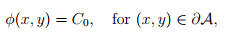(9. 49 )

where Cis a constant. Since, the stress and displacement fields depend only in the derivative of the stress potential and not its value at a location, it suﬃces to ﬁnd this stress function up to a constant2. Therefore, we arbitrarily set C0 = 0, understanding that it can take any value and that the stress and displacement ﬁeld would not change because of this. Hence, equation (9.49) reduces to requiring,

φ(x,y) = 0, for (x,y) ∈ ∂A.                                 (9.50)

The boundary condition (9.21) and (9.22) for the stress state (9.44) require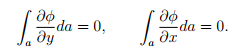(9.51)

Appealing to Green’s theorem for simply connected domains, the above equation (9.51) reduces to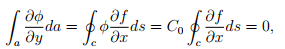(9.52)(9.53)

where we have used (9.49) and the fact thatHc ∂f ∂xds = 0 andHc ∂f ∂yds = 0 for closed curves.

Finally, the boundary conditions (9.23) and (9.24) for the stress state (9.44) yields,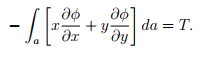(9.54)

Noting that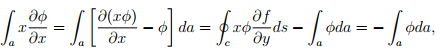(9.55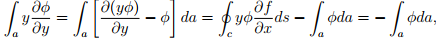(9.56)

where we have used the Green’s theorem for simply connected domain and equation (9.50). Substituting the above equations in (9.54) we obtain,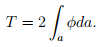(9.57)

 2This means that if φ is a stress function for the given boundary value problem then φ+C0, where C0 is some constant, will also be an admissible stress function for the same boundary value problem.

Thus, we have to ﬁnd φ such that the governing equation (9.47) has to hold along with the boundary condition (9.50). Then, we use equation (9.57) to ﬁnd the torsional moment required to realize a given angle of twist per unit length Ω.

Membrane analogy

The governing equation (9.47) along with the boundary condition (9.50) and (9.57) is identical with those governing the static deﬂection under uniform pressure of an elastic membrane in the same shape as that of the member subjected to torsion. This fact creates an analogy between the torsion problem and elastic membrane under uniform pressure. This analogy is exploited to get the qualitative features that the stress function should posses and this aids in developing approximate solutions. Obtaining the governing equation for the static deﬂection of an elastic membrane under uniform pressure and showing that it is identical to (9.47) is beyond the scope of this lecture notes. One may refer Sadd for the same.

Offer running on EduRev: Apply code STAYHOME200 to get INR 200 off on our premium plan EduRev Infinity!

## Advanced Solid Mechanics - Notes, Videos, MCQs & PPTs

40 videos|61 docs

,

,

,

,

,

,

,

,

,

,

,

,

,

,

,

,

,

,

,

,

,

;